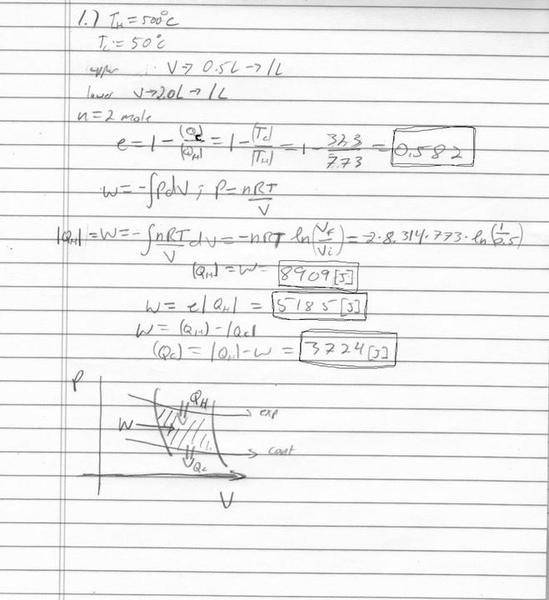# A Carnot engine. Got right answers, but I have questions on solution.

## Homework Statement

A Carnot engine runs between a high temperature of 500 C and a low temperature of 50 C. The isothermal expansion (upper branch) occurs between a volume of 0.5L and 1.0L. The isothermal contraction (lower branch) occurs between 2.0L and 1.0L. There are 2 moles of gas involved. Calculate the work done by the engine, the heat taken in, and the heat ejected. calculate the efficiency o the cycle. Draw a PV diagram and show the area corresponding to the total work being done. Show the beginning and end points of the isothermal and adiabatic curves. Show during which branch of the cycle heat enters and leaves the engine.

## The Attempt at a Solution

So why does QH = -W, but it also equals QC + W ?
And W=e*QH? With e = efficiency

Hmm. Ok so when I typed that, something sparked. So I said QH is = to W. But then I actually calculated the work for my answer based off of QH*e. I just calculated the work for the lower branch and it came out to equal QC.

So how can I make sure not to confuse myself I guess? The heat entering the system is work being done on the system and work leaving is work being done BY the system? So then I can find the total work of the system which may or may not be positive?

Why doesn't the total W=e*QC?
Thanks for the help## Answers and Replies

rock.freak667
Homework Helper
In terms of the definition of efficiency

$$\eta = \frac{Energy \ Output}{Energy \ Input} = \frac{W}{Q_H}$$

But if you apply the first law of thermodynamics to the cycle ∑Q=∑W which comes out as

QH-QC=W ⇒QH=QC+W

OR the energy supplied = work done by engine + heat rejected by engine

EDIT: Also in your working for QH, isn't that an adiabatic expansion line?

Hey thanks! So, what Work equals $$- \int PDV$$ ?

EDIT: Also in your working for QH, isn't that an adiabatic expansion line?
Well, to tell you the truth I'm not quite sure what you're asking. I'm sure the adiabatic lines are the vertical ones.

rock.freak667
Homework Helper
In general W=∫ P dV

But in an adiabatic process PVγ = Constant, so the P will change

Isothermal process PV= Constant, so the P will change here.

So the work done in each process uses a different formula.

In general W=∫ P dV

But in an adiabatic process PVγ = Constant, so the P will change

Isothermal process PV= Constant, so the P will change here.

So the work done in each process uses a different formula.

Ok thanks! So just to clarify, did you mean to say P will change in both cases?

rock.freak667
Homework Helper
Ok thanks! So just to clarify, did you mean to say P will change in both cases?

What I meant was, that depending on the type of process, the end result for the work done will depend on different parameters, not just in all cases it will be W=nRT ln(V2/V1)

Isn't an adiabatic process a process in which no heat is transferred to or from the system? How can this be an adiabatic process if heat is transferred?

And when you say PV=constant, so P will change. Do you mean that PV is constant and since V changes, P much change to accommodate the change in V.

Last edited:
Andrew Mason
Science Advisor
Homework Helper
Isn't an adiabatic process a process in which no heat is transferred to or from the system? How can this be an adiabatic process if heat is transferred?
The reference is to a part of the cycle. The four-part Carnot cycle has two parts that are isothermal and two that are adiabatic. The heat flow into or out of the engine occurs only during the isothermal parts.

And when you say PV=constant, so P will change. Do you mean that PV is constant and since V changes, P must change to accommodate the change in V.
Correct.

AM